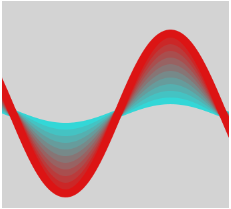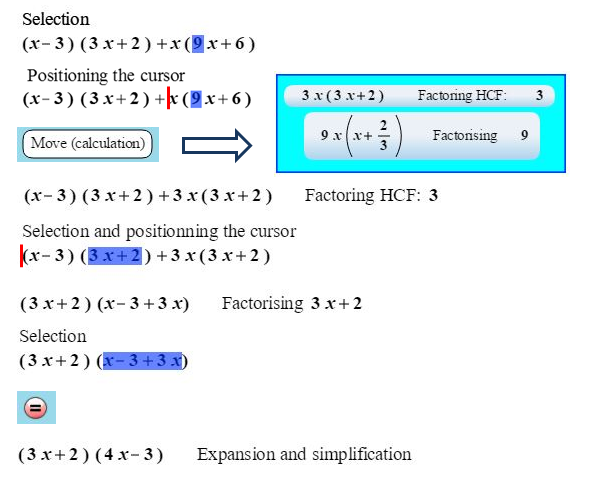﻿ EpsilonWriter ﻿
EpsilonWriter Web

# EpsilonWriter Web for computers, tablets and smartphones

## An application to draw and animate functions and curves

### Drawing curves

Drawing functions, parametric or polar curves, choosing color, thickness and zoom.### Animated curves

Animating curves (translation, rotation, zoom, distortion) with persistence and colors gradient.## A dynamic algebra application to do calculations by steps and with explanations

• Calculations by selection and move (a variant of the drag and drop adapted to touch use for tablets and smartphones) : you select an expression then you put the cursor where you want and ask for a "Move (Calculation)". EpsilonWriter proposes transformations when the situation allows them. The gestures are transformed to mathematical operations:
• numerical calculations, expand and simplify,
• factoring, simplification,
• adding to the 2 members (substraction, multiplication, division), substitution.
• Calculations on selection : you select an expression the you ask for a calculation. EpsilonWriter proposes an expression then you ask for a calculation. EpsilonWriter proposes calculations when the situation allows them :
• Exact or approximate numerical calculations, expand and simplify,
• Second and third degree equations solving schemes,
• Transform some logical conditions to a domain or a set of solutions,
• Limit calculation, derivative calculation,
• Table of signs, Table of variations,
• Formula generation,
• Complex numbers calculations.## An application to run resources with :

• Questions with a single or several choices
• Calculations to do with results that are mathematically checked
• Identity to complete
• Several mathematical representation to give
When the student ask for questions checking, she get a score and an explanation.

# EpsilonWriter Creator for computers Windows, MacOS, Linux

## A document and pedagogical resources editor

Write easily documents with formulas.

• Enter and modify formulas very easily.
• Copy from Wikipédia and paste to EpsilonWriter : the formulas you got are editable.
• Import Latex.
• Print EpsilonWriter documents, course or exercise sheets
• Export web pages in HTML with images for the formulas and in XHTML with MathML formulas.
• Use EpsilonWriter for your emails and blogs

Create Math questionnaires

• Write questions with single or several answers.
• Write questions with an open mathematical answer.
• Write comments that appear during the assessment.

Create easily innovative and playful resources, for computers, tablets and smartphones.

• TQuiz games
• Aplusix Neo interactive exercises

Publish your resources with your online workspace and on the epsilon-puli.net website

• Register to epsilon-publi.net and get a free online storage space for your resources. You can access this space directly from the EpsilonWriter's web explorer
• You can publish your resources in the catalog of the resources of the website.
• You can discover and use other resources on the website

The Aristod company, which developed these tools, ceased its activities in April 2019, due to the very low interest that these tools have generated.
Jean-Francois Nicaud, the main author of these tools, keeps them available to users on this website for a few years.

Contact: jeanfrancois dot nicaud at laposte dot net